Author: Oscar Cronquist Article last updated on September 06, 2022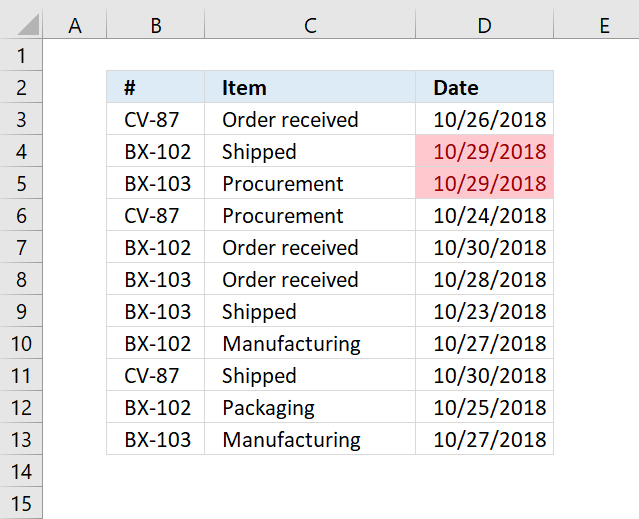Excel has a built-in feature that allows you to highlight dates if a given condition is met. Section 1 below demonstrates how to create a conditional formatting rule highlighting cells based on date values using the built-in tools. The drop-down list in the dialog box lets you pick from a variety of date conditions:

Yesterday | Today | Tomorrow | In the last 7 days | Last week | This week | Next week | Last month | This month | Next month

Section 2 shows how to create conditional formatting formulas highlighting rows or records in a data set also based on a condition evaluating date values. I will also explain how these conditional formatting formulas work in great detail.

## 1. How to apply Conditional Formatting

1. Select cell range containing dates.
2. Go to tab "Home" on the ribbon if you are not already there.
3. Press with left mouse button on "Conditional formatting" button.
4. Press with mouse on "Highlight Cells Rules".
5. Press with left mouse button on "A Date Occuring..."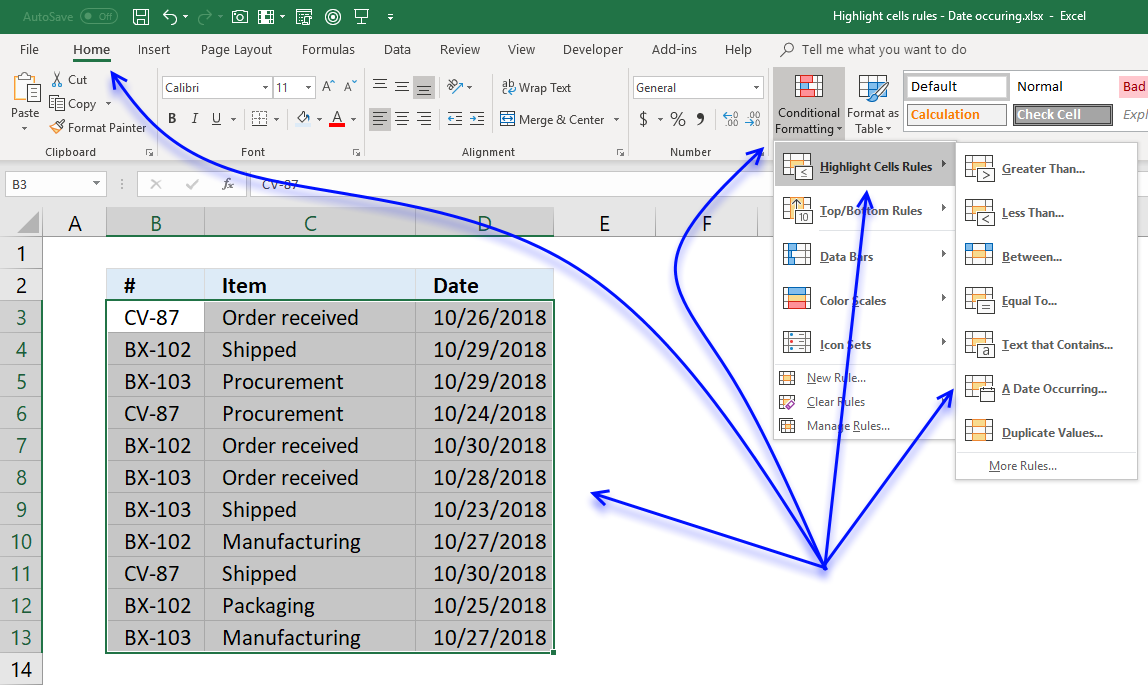6. A dialog box appears that lets you specify the date condition and the formatting.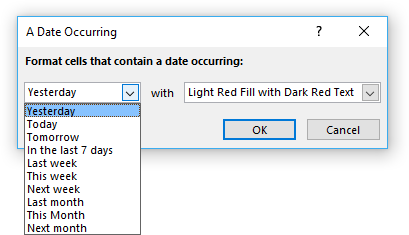7. Pick a prebuilt formatting or use custom format to create a new one.
8. Press with left mouse button on OK button.

## 2. Highlight rows/records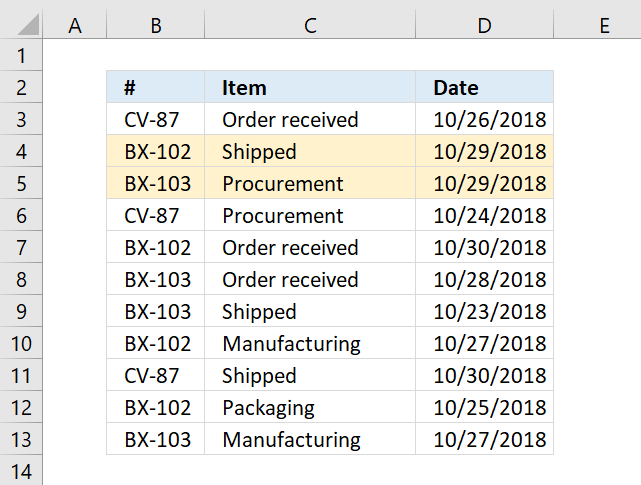You need to use a formula instead of the prebuilt ones in order to highlight the entire row if the date meets the condition.

1. Go to tab "Home" on the ribbon.
2. Press with left mouse button on the "Conditional Formatting" button.
3. Press with left mouse button on "New Rule.." to open a dialog box.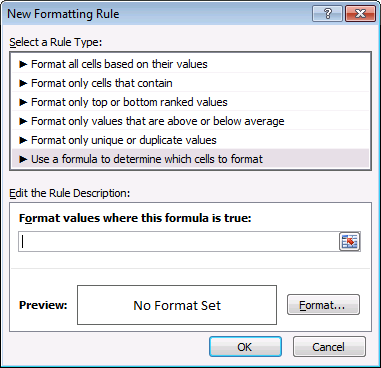4. Press with left mouse button on "Use a formula to determine which cells to format".
5. Type the formula. (See below which formula to use).
6. Press with left mouse button on "Format..." button and choose a formatting.
7. Press with left mouse button on OK button twice.

## 2.1 Highlight a row if the date is yesterday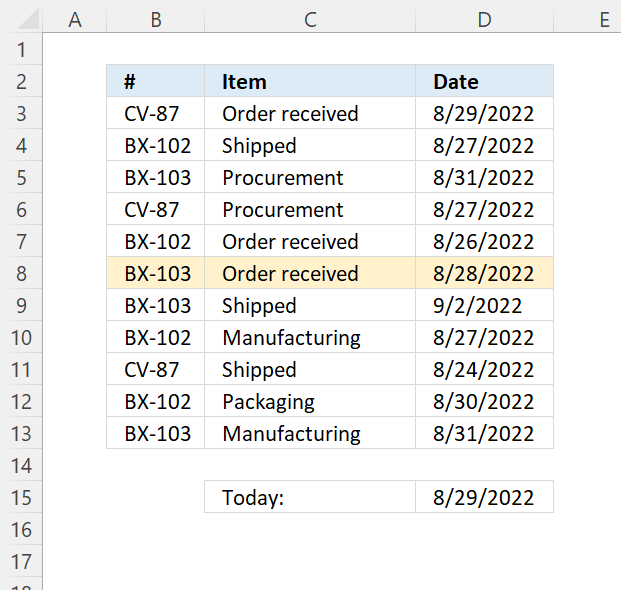The following formula highlights all cells on the same row if the date in column D is yesterday:

=\$D3=(TODAY()-1)

The \$ (dollar sign) makes the cell reference absolute meaning it locks the column thus highlighting all cells on the same row if the date matches the condition.

Read section 2 for instructions on how to insert a conditional formatting formula.

### Explaining CF formula in cell D3

#### Step 1 - Calculate today's date

The TODAY function returns the Excel date (serial number) of the current date.

Function syntax: TODAY()

The TODAY() function is volatile meaning it recalculates more often than regular formulas, this may slow down worksheet calculations.

TODAY()

returns

8/29/2022

#### Step 2 - Calculate yesterday

The minus sign lets you subtract numbers in an Excel formula.

TODAY()-1

becomes

44802 (8/29/2022)

and returns 44801 (8/28/2022).

#### Step 3 - Compare yesterday to dates in D3 and cells below

\$D3 is both a relative and absolute cell reference, the dollar sign makes D absolute and row number three is a relative cell reference.

This changes the Conditional Formatting formula when a new cell is evaluated, in other words, the row or record is highlighted if the condition is met.

The equal sign lets you compare value to value, the parentheses control the order of operation. We need to calculate yesterday's date before we compare the date numbers.

\$D3=(TODAY()-1)

becomes

44802=44801

and returns FALSE. Cell D3 is not highlighted, in fact, no cells on row three are highlighted.

## 2.2 Highlight a row if the date is today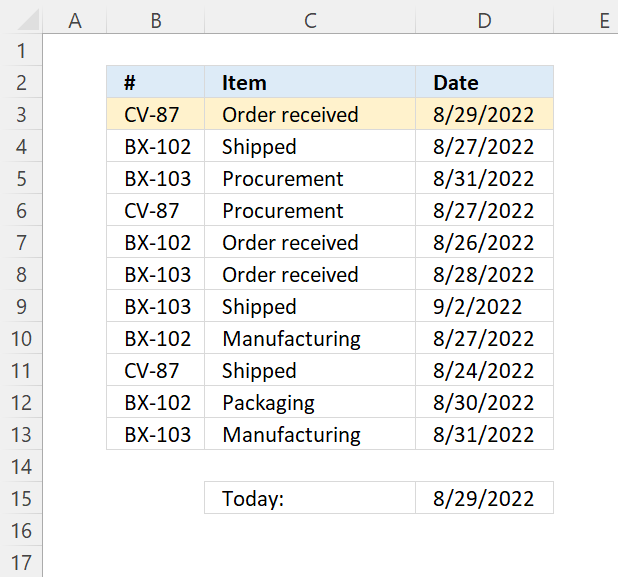Conditional formatting formula:

=\$D3=TODAY()

Read section 2 for instructions on how to insert a conditional formatting formula.

### Explaining formula

Read section 2.1 Highlight a row if the date is yesterday above for an explanation.

## 2.3 Highlight a row if the date is tomorrow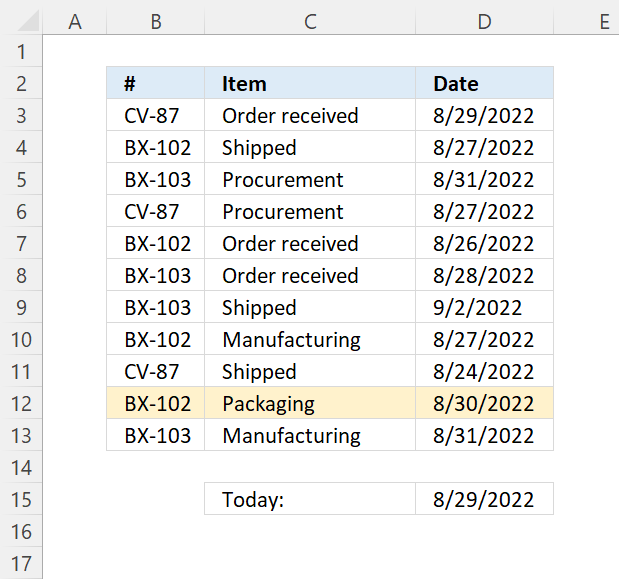Conditional formatting formula:

=\$D3=(TODAY()+1)

Read section 2 for instructions on how to insert a conditional formatting formula.

### Explaining CF formula in cell D3

#### Step 1 - Calculate today's date

The today function returns the Excel date (serial number) of the current date.

Function syntax: TODAY()

The TODAY() function is volatile meaning it recalculates more often than regular formulas, this may slow down worksheet calculations.

TODAY()

returns

8/29/2022

#### Step 2 - Calculate yesterday

The plus sign lets you add numbers in an Excel formula.

TODAY()+1

becomes

44802 (8/29/2022)

and returns 44803 (8/30/2022).

#### Step 3 - Compare yesterday to dates in D3 and cells below

\$D3 is both a relative and absolute cell reference, the dollar sign makes D absolute and row number three is a relative cell reference.

This changes the Conditional Formatting formula when a new cell is evaluated, in other words, the row or record is highlighted if the condition is met.

The equal sign lets you compare value to value, the parentheses control the order of operation. We need to calculate yesterday's date before we compare the date numbers.

\$D3=(TODAY()-1)

becomes

44802=44803

and returns FALSE. Cell D3 is not highlighted, in fact, no cells on row three are highlighted.

## 2.4 Highlight a row if the date is in the last 7 days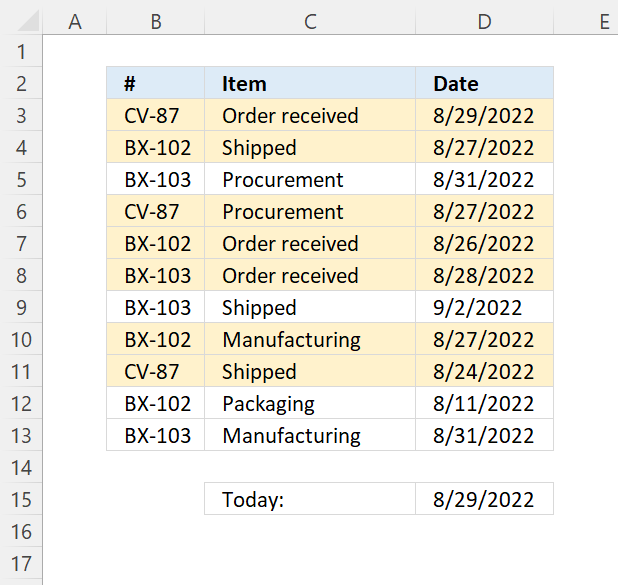=(\$D3>=(TODAY()-7))*(\$D3<=TODAY())

Read section 2 for instructions on how to insert a conditional formatting formula.

### Explaining CF formula in cell D3

#### Step 1 - Calculate today's date

The today function returns the Excel date (serial number) of the current date.

Function syntax: TODAY()

The TODAY() function is volatile meaning it recalculates more often than regular formulas, this may slow down worksheet calculations.

TODAY()

returns

44802 (8/29/2022).

#### Step 2 - Calculate seven days back

TODAY()-7

becomes

44802-7

and returns

44795 (8/22/2022).

#### Step 3 - Check if the date in cell \$D3 is equal or larger than seven days back counting from today

The larger than, smaller than, and the equal sign are logical operators, they return TRUE if the condition is met and FALSE if not.

\$D3>=(TODAY()-7)

becomes

44802>=44795

and returns TRUE.

#### Step 4 - Check if the date in \$D3 is smaller or equal to today's date

\$D3<=TODAY()

becomes

44802>=44802

and returns TRUE.

#### Step 5 - Apply AND logic, both conditions must be true

The asterisk character lets you multiply numbers and boolean values in an Excel formula, this applies AND logic to boolean values.

TRUE * TRUE = TRUE
TRUE * FALSE = FALSE
FALSE * FALSE = FALSE

This means that both values must be TRUE to return TRUE (AND logic).

The parentheses control the order of operation, we need to compare values before we multiply.

(\$D3>=(TODAY()-7))*(\$D3<=TODAY())

becomes

(TRUE)*(TRUE)

and returns 1. Boolean values are converted to their numerical equivalents. TRUE - 1, FALSE - 0 (zero).

## 2.5 Highlight a row if the date is in the last week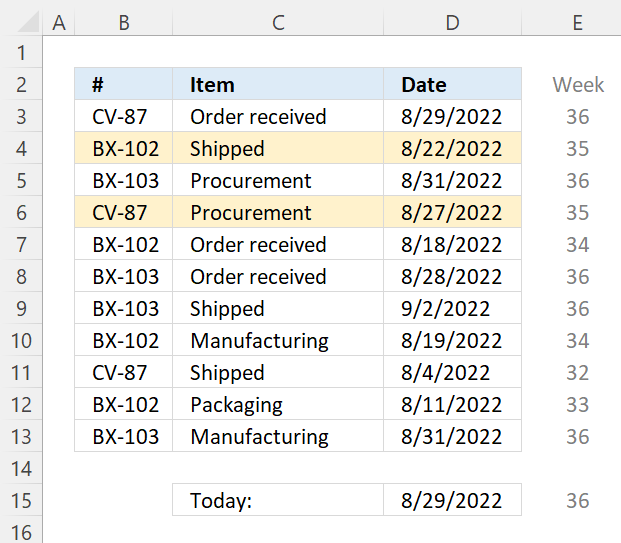=WEEKNUM(\$D3,1)=(WEEKNUM(TODAY(),1)-1)

Change the second argument in WEEKNUM function if the week doesn't begin with Sunday.

Read section 2 for instructions on how to insert a conditional formatting formula.

### Explaining CF formula in cell D3

#### Step 1 - Calculate today's date

The today function returns the Excel date (serial number) of the current date.

Function syntax: TODAY()

The TODAY() function is volatile meaning it recalculates more often than regular formulas, this may slow down worksheet calculations.

TODAY()

returns

44802 (8/29/2022).

#### Step 2 - Calculate the current week's number

The WEEKNUM function calculates a given date's week number based on a return_type parameter that determines which day the week begins.

Function syntax: WEEKNUM(serial_number,[return_type])

WEEKNUM(TODAY(),1)

becomes

WEEKNUM(44802,1)

and returns 36.

#### Step 3 - Calculate the last week's number

The minus sign lets you subtract numbers in an Excel formula.

WEEKNUM(TODAY(),1)-1

becomes

36-1

and returns 35.

#### Step 4 - Check if week's number is equal to last week's number

The larger than, smaller than, and the equal sign are logical operators, they return TRUE if the condition is met and FALSE if not.

WEEKNUM(\$D3,1)=(WEEKNUM(TODAY(),1)-1)

becomes

36=35

and returns FALSE. Row 3 is not highlighted.

## 2.6 Highlight a row if the date is in this week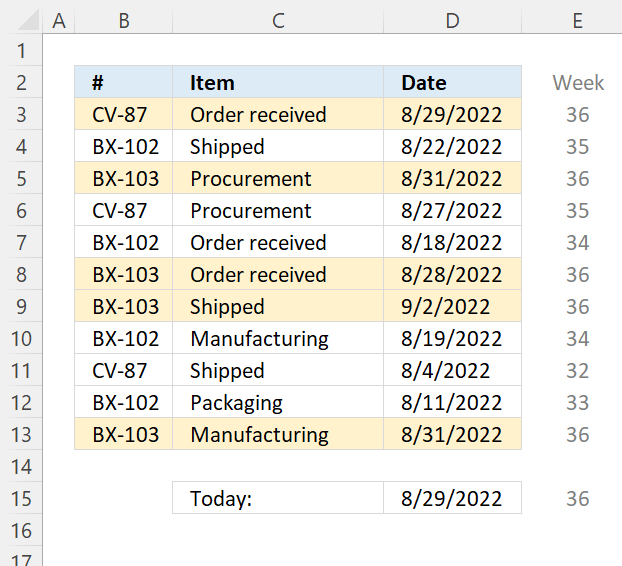=WEEKNUM(\$D3,1)=WEEKNUM(TODAY(),1)

Change the second argument in WEEKNUM function if the week doesn't begin with Sunday.

Read section 2 for instructions on how to insert a conditional formatting formula.

### Explaining CF formula in cell D3

#### Step 1 - Calculate today's date

The today function returns the Excel date (serial number) of the current date.

Function syntax: TODAY()

The TODAY() function is volatile meaning it recalculates more often than regular formulas, this may slow down worksheet calculations.

TODAY()

returns

44802 (8/29/2022).

#### Step 2 - Calculate the current week's number

The WEEKNUM function calculates a given date's week number based on a return_type parameter that determines which day the week begins.

Function syntax: WEEKNUM(serial_number,[return_type])

WEEKNUM(TODAY(),1)

becomes

WEEKNUM(44802,1)

and returns 36.

#### Step 3 - Check if week's number is equal to last week's number

The larger than, smaller than, and the equal sign are logical operators, they return TRUE if the condition is met and FALSE if not.

WEEKNUM(\$D3,1)=(WEEKNUM(TODAY(),1))

becomes

36=36

and returns TRUE. Row 3 is not highlighted.

## 2.7 Highlight a row if the date is in the next week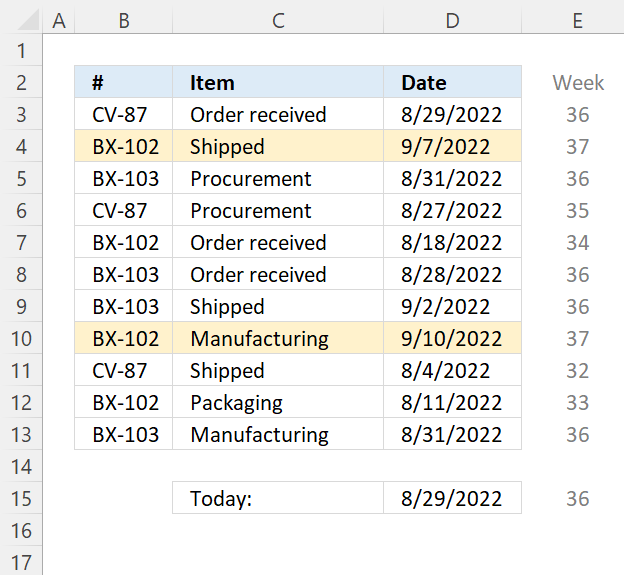=WEEKNUM(\$D3,1)=(WEEKNUM(TODAY(),1)+1)

Change the second argument in WEEKNUM function if the week doesn't begin with Sunday.

Read section 2 for instructions on how to insert a conditional formatting formula.

### Explaining CF formula in cell D3

#### Step 1 - Calculate today's date

The TODAY function returns the Excel date (serial number) of the current date.

Function syntax: TODAY()

The TODAY() function is volatile meaning it recalculates more often than regular formulas, this may slow down worksheet calculations.

TODAY()

returns

44802 (8/29/2022).

#### Step 2 - Calculate the current week's number

The WEEKNUM function calculates a given date's week number based on a return_type parameter that determines which day the week begins.

Function syntax: WEEKNUM(serial_number,[return_type])

WEEKNUM(TODAY(),1)

becomes

WEEKNUM(44802,1)

and returns 36.

#### Step 3 - Calculate the last week's number

The plus sign lets you add numbers in an Excel formula.

WEEKNUM(TODAY(),1)+1

becomes

36-1

and returns 35.

#### Step 4 - Check if week's number is equal to last week's number

The larger than, smaller than, and the equal sign are logical operators, they return TRUE if the condition is met and FALSE if not.

WEEKNUM(\$D3,1)=(WEEKNUM(TODAY(),1)-1)

becomes

36=35

and returns FALSE. Row 3 is not highlighted.

## 2.8 Highlight a row if the date is in the last month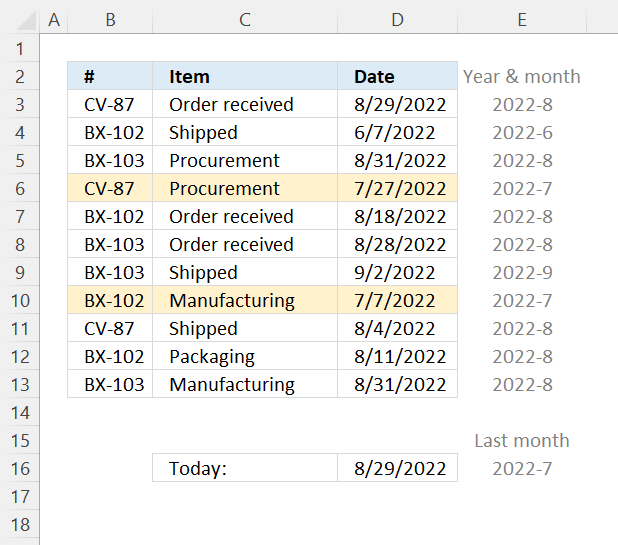=TEXT(\$D3,"YYYY-M")=(YEAR(TODAY())&"-"&MONTH(TODAY())-1)

Read section 2 for instructions on how to insert a conditional formatting formula.

### Explaining CF formula in cell D3

#### Step 1 - Calculate today's date

The TODAY function returns the Excel date (serial number) of the current date.

Function syntax: TODAY()

TODAY()

returns

44802 (8/29/2022).

#### Step 2 - Calculate today's year

The YEAR function converts a date to a number representing the year in the date.

Function syntax: YEAR(serial_number)

YEAR(TODAY())

becomes

YEAR(44802)

and returns 2022.

#### Step 3 - Calculate today's month number

The MONTH function extracts the month as a number from an Excel date.

Function syntax: MONTH(serial_number)

MONTH(TODAY())

becomes

MONTH(44802)

and returns 8.

#### Step 4 - Subtract month number by 1

To get last month we need to subtract the month number by 1. The minus character lets you subtract numbers in an Excel formula.

MONTH(TODAY())-1

becomes

8-1

and returns 7.

#### Step 5 - Concatenate strings

The ampersand character lets you merge strings in an Excel formula.

YEAR(TODAY())&"-"&MONTH(TODAY())-1

becomes

2022&"-"&7

and returns 2022-07

#### Step 6 - Calculate year and month from date

The TEXT function converts a value to text in a specific number format.

Function syntax: TEXT(value, format_text)

TEXT(\$D3,"YYYY-M")

becomes

TEXT(44802,"YYYY-M")

and returns

2022-8

#### Step 7 - Compare strings

The equal sign lets you compare values in an Excel formula, the result is a boolean value TRUE or FALSE.

TEXT(\$D3,"YYYY-M")=(YEAR(TODAY())&"-"&MONTH(TODAY())-1)

becomes

"2022-8"="2022-7"

and returns FALSE. Cell D3 is not highlighted.

## 2.9 Highlight a row if the date is in this month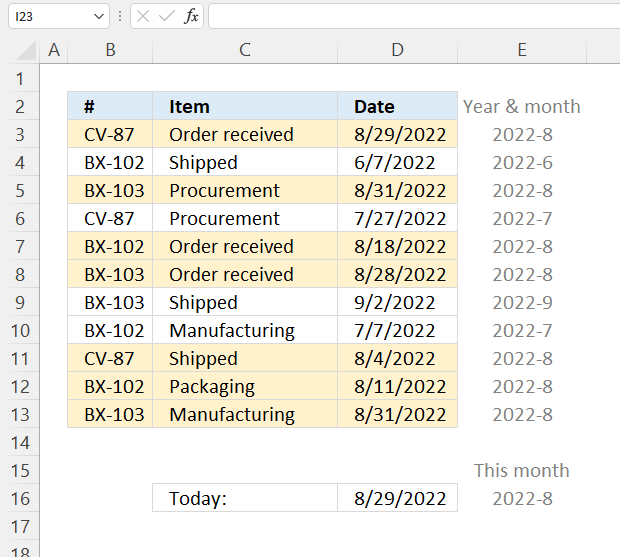=TEXT(\$D3, "YYYY-M")=TEXT(TODAY(), "YYYY-M")

Read section 2 for instructions on how to insert a conditional formatting formula.

### Explaining CF formula in cell D3

#### Step 1 - Calculate today's date

The TODAY function returns the Excel date (serial number) of the current date.

Function syntax: TODAY()

TODAY()

returns

44802 (8/29/2022).

#### Step 2 - Calculate today's year and month

The TEXT function converts a value to text in a specific number format.

Function syntax: TEXT(value, format_text)

TEXT(TODAY(), "YYYY-M")

becomes

TEXT(44802,"YYYY-M")

and returns

2022-8.

#### Step 3 - Calculate cell D3's year and month

The TEXT function converts a value to text in a specific number format.

Function syntax: TEXT(value, format_text)

TEXT(\$D3, "YYYY-M")

becomes

TEXT(44802,"YYYY-M")

and returns

2022-8.

#### Step 4 - Compare year and month

The equal sign lets you compare values in an Excel formula, the result is a boolean value TRUE or FALSE.

TEXT(\$D3, "YYYY-M")=TEXT(TODAY(), "YYYY-M")

becomes

"2022-8"="2022-8"

and returns TRUE. Cell D3 is highlighted.

## 2.10 Highlight a row if the date is in the next month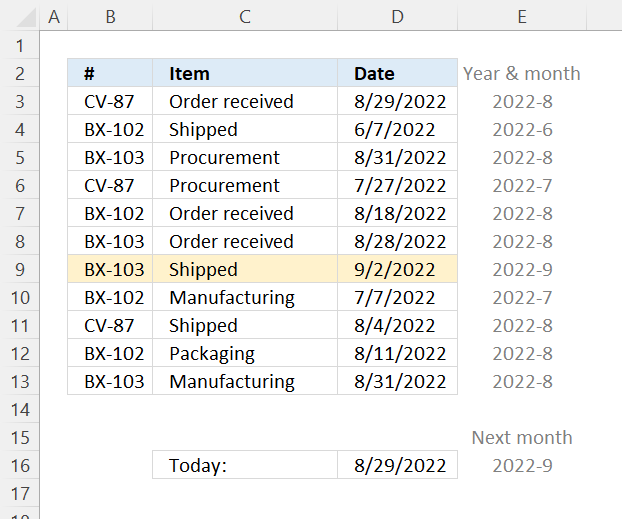=TEXT(\$D3,"YYYY-M")=(YEAR(TODAY())&"-"&MONTH(TODAY())+1)

Read section 2 for instructions on how to insert a conditional formatting formula.

### Explaining CF formula in cell D3

#### Step 1 - Calculate today's date

The TODAY function returns the Excel date (serial number) of the current date.

Function syntax: TODAY()

TODAY()

returns

44802 (8/29/2022).

#### Step 2 - Calculate today's year

The YEAR function converts a date to a number representing the year in the date.

Function syntax: YEAR(serial_number)

YEAR(TODAY())

becomes

YEAR(44802)

and returns 2022.

#### Step 3 - Calculate today's month number

The MONTH function extracts the month as a number from an Excel date.

Function syntax: MONTH(serial_number)

MONTH(TODAY())

becomes

MONTH(44802)

and returns 8.

#### Step 4 - Add 1 to the month number

To get next month we need to add 1 to the month number. The plus character lets you add numbers in an Excel formula.

MONTH(TODAY())+1

becomes

8+1

returns 9.

#### Step 5 - Concatenate strings

The ampersand character lets you merge strings in an Excel formula.

YEAR(TODAY())&"-"&MONTH(TODAY())+1

becomes

2022&"-"&9

and returns

"2022-9".

#### Step 6 - Calculate year and month from date

The TEXT function converts a value to text in a specific number format.

Function syntax: TEXT(value, format_text)

TEXT(\$D3,"YYYY-M")

becomes

TEXT(44802,"YYYY-M")

and returns

"2022-8".

#### Step 7 - Compare strings

TEXT(\$D3,"YYYY-M")=(YEAR(TODAY())&"-"&MONTH(TODAY())+1)

becomes

"2022-8"="2022-9"

and returns FALSE.